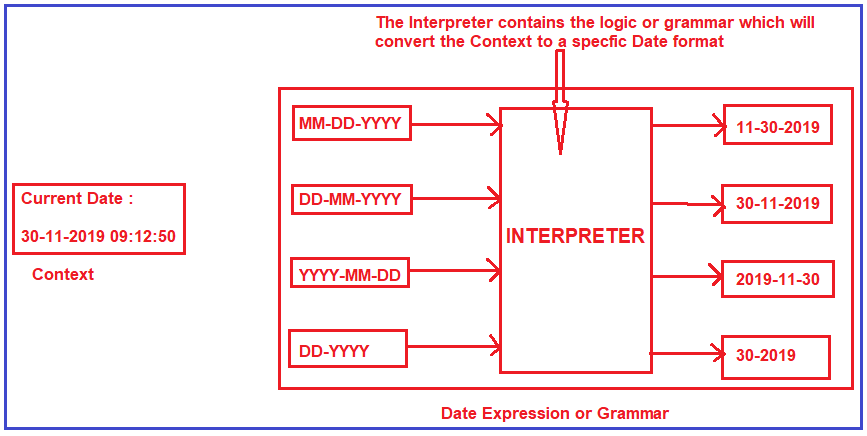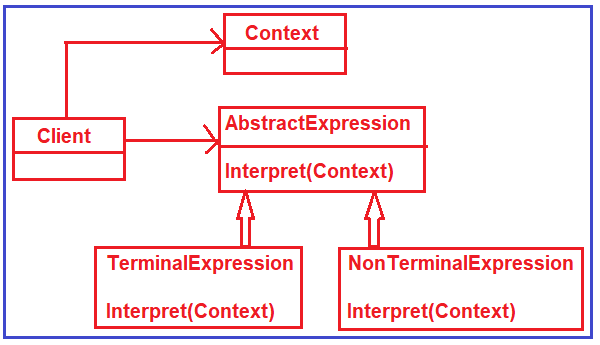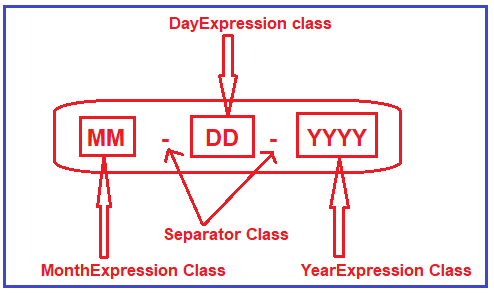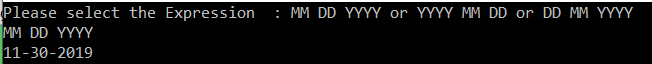# Interpreter Design Pattern in C#

## Interpreter Design Pattern in C#

In this article, I am going to discuss the Interpreter Design Pattern in C# with examples. Please read our previous article where we discussed the Strategy Design Pattern in C# with real-time examples. The Interpreter Design Pattern falls under the category of Behavioural Design Pattern. As part of this article, we are going to discuss the following pointers in detail.

1. What is the Interpreter Design Pattern?
2. Understanding the Class Diagram of Interpreter Design Pattern
3. Implementing the Interpreter Design Pattern in C#.
4. Real-time Examples of Interpreter Design Pattern
5. When to use the Interpreter Design Pattern?
##### What is the Interpreter Design Pattern?

The Interpreter Design Pattern Provides a way to evaluate language grammar or expression. This pattern is used in SQL parsing, symbol processing engine, etc.

Let us understand the interpreter design pattern with an example. Please have a look at the following image. On the left-hand side, you can see the Context. The Context is nothing but the value that we want to interpret. Here, the context value is the current date. On the right-hand side, you can see the Date expression or you can say the grammar. We have different types of date expression such as (MM-DD-YYYY, DD-MM-YYYY, YYYY-MM-DD, and DD-YYYY).Suppose, you want the date in MM-DD-YYYY format then what you need to do is, you need to pass the Context value and the Date Expression you want (i.e. MM-DD-YYYY) to the interpreter. What the interpreter will do is, it will convert the context value into the date expression format you passed to it. So, basically the interpreter contains the logic or grammar to convert the context object into a specific readable format.

##### Understanding the Class Diagram of Interpreter Design Pattern:

In order to understand the class diagram, please have a look at the following image.Context: This is a class that contains the data that we want to interpret.

AbstractExpression: This is either an interface that defines the interpret method which should be implemented by the subclasses. This method takes the context object as a parameter. This context object holds the data that we want to interpret.

TerminalExpression: This is a concrete class that implements the Interpret abstract method of the AbstractExpression interface. The Terminal Expression represents elements in the grammar that do not get replace such as symbols.

NonTerminalExpression: This is also a concrete class implements the AbstractExpression interface. The NonTerminalExpression represents elements that will be replaced during the evaluation such as variables or even rules.

Client: This is a class that builds the abstract syntax tree for a set of instructions in the given grammar. This tree builds with the help of instances of NonTerminalExpression and TerminalExpression classes.

##### Implementation of Interpreter Design Pattern in C#:

Let us implement the example that we discussed here step by step using the Interpreter Design Pattern in C#. So, here we need to convert date time to a specific format. In order to achieve this, we can define different types of grammar. Please have a look at the following diagram. As you can see in the following image, we define a class for each type of grammar such as Month, Year, Day and separator. So, using this grammar you can create any type of date format##### Step1: Creating Context

Create a class file with the name Context.cs and then copy and paste the following code in it. This class contains the date (i.e. current date-time) that we want to interpret.

```using System;
namespace InterpreterDesignPattern
{
public class Context
{
public string expression { get; set; }
public DateTime date { get; set; }
public Context(DateTime date)
{
this.date = date;
}
}
}
```
##### Step2: Creating AbstractExpression

Create an interface with the name AbstractExpression and then copy and paste the following code in it. This class defines the method that is going to be implemented by the child classes. Moreover, the method takes the Context object as a parameter. This context object holds the value that we want to interpret.

```namespace InterpreterDesignPattern
{
public interface AbstractExpression
{
void Evaluate(Context context);
}
}
```
##### Step3: Terminal Expressions

These are going to be the concrete classes that implement the AbstractExpression interface. Each class implements the Evaluate method according to its own grammar.

###### DayExpression:

Create a class file with the name DayExpression and then copy and paste the following code. The evaluate method replaces the expression DD value with the exact Day value that is stored in the context object.

```namespace InterpreterDesignPattern
{
public class DayExpression : AbstractExpression
{
public void Evaluate(Context context)
{
string expression = context.expression;
context.expression = expression.Replace("DD", context.date.Day.ToString());
}
}
}
```
###### MonthExpression:

Create a class file with the name MonthExpression and then copy and paste the following code. The evaluate method replaces the expression MM value with the exact Month value that is stored in the context object.

```namespace InterpreterDesignPattern
{
public class MonthExpression : AbstractExpression
{
public void Evaluate(Context context)
{
string expression = context.expression;
context.expression = expression.Replace("MM", context.date.Month.ToString());
}
}
}
```
###### YearExpression:

Create a class file with the name YearExpression and then copy and paste the following code. The evaluate method replaces the expression YYYY value with the exact Year value that is stored in the context object.

```namespace InterpreterDesignPattern
{
public class YearExpression : AbstractExpression
{
public void Evaluate(Context context)
{
string expression = context.expression;
context.expression = expression.Replace("YYYY", context.date.Year.ToString());
}
}
}

```
###### SeparatorExpression:

Create a class file with the name YearExpression and then copy and paste the following code. The evaluate method simply replace the space with a hyphen (-) separator.

```namespace InterpreterDesignPattern
{
class SeparatorExpression : AbstractExpression
{
public void Evaluate(Context context)
{
string expression = context.expression;
context.expression = expression.Replace(" ", "-");
}
}
}
```

Note: In this example, we are not using the NonTerminalExpression.

##### Step4: Client

Modify the Main method as shown below. This is going to be our client code. Here, we will ask the user to select the date format and then we will display the date as per the user-selected date format.

```using System;
using System.Collections.Generic;

namespace InterpreterDesignPattern
{
class Program
{
static void Main(string[] args)
{
List<AbstractExpression> objExpressions = new List<AbstractExpression>();
Context context = new Context(DateTime.Now);
Console.WriteLine("Please select the Expression  : MM DD YYYY or YYYY MM DD or DD MM YYYY ");

string[] strArray = context.expression.Split(' ');

foreach(var item in strArray)
{
if(item == "DD")
{
}
else if (item == "MM")
{
}
else if (item == "YYYY")
{
}
}

foreach(var obj in objExpressions)
{
obj.Evaluate(context);
}

Console.WriteLine(context.expression);
}
}
}
```

Output:##### Real-time Examples of Interpreter Design Pattern:

The best example of this pattern is C# Compiler (CSC) that interprets the C# Source code into byte code that is understood by CLR.

Google Translator is an example of the interpreter design pattern where the input can be in any language and we get the output in another language.

##### When to use the Interpreter Design Pattern?

The Interpreter Design Pattern should be used when there is a language to interpret. The interpreter pattern works best when

1. Grammar is simple
2. Efficiency is not a critical concern

In the next article, I am going to discuss the Mediator Design Pattern in C# with examples. Here, in this article, I try to explain the Interpreter Design Pattern step by step with an example. I hope you understood the need and use of the Interpreter Design Pattern in C#.

### 1 thought on “Interpreter Design Pattern in C#”

1.and this code is true:
//foreach (var item in strArray)
//{
// if (item == “DD”)
// {
// }
// else if (item == “MM”)
// {
// }
// else if (item == “YYYY”)
// {